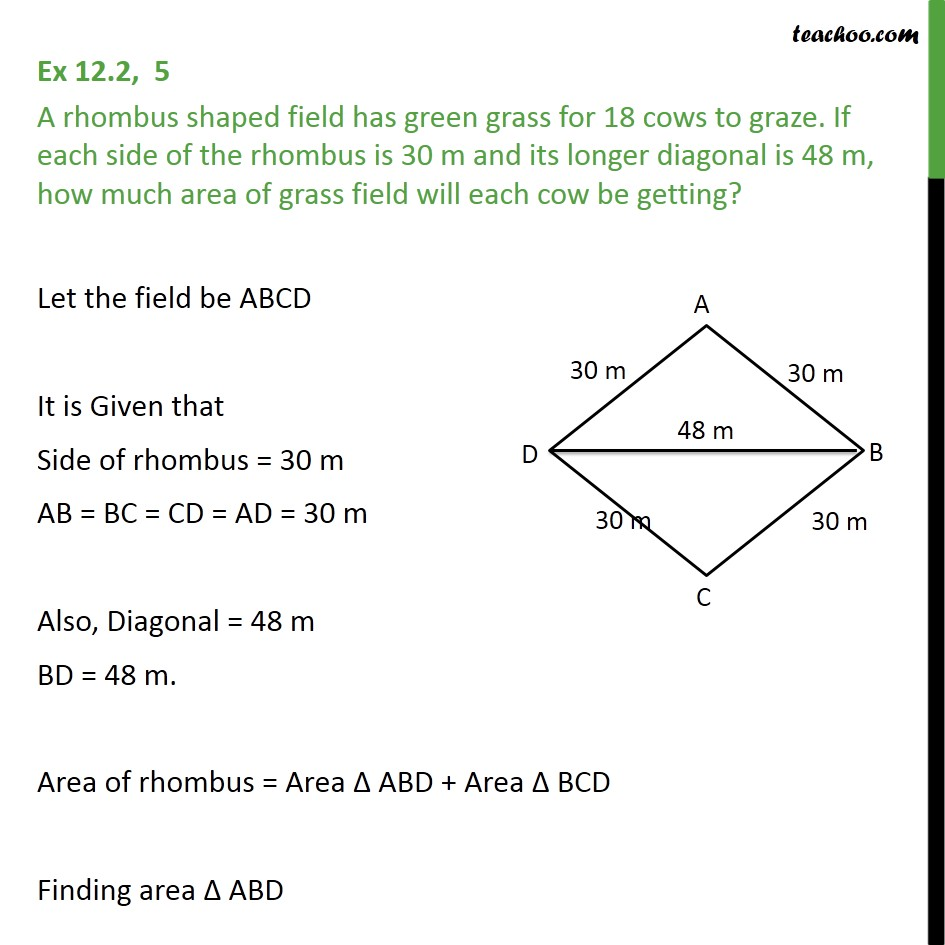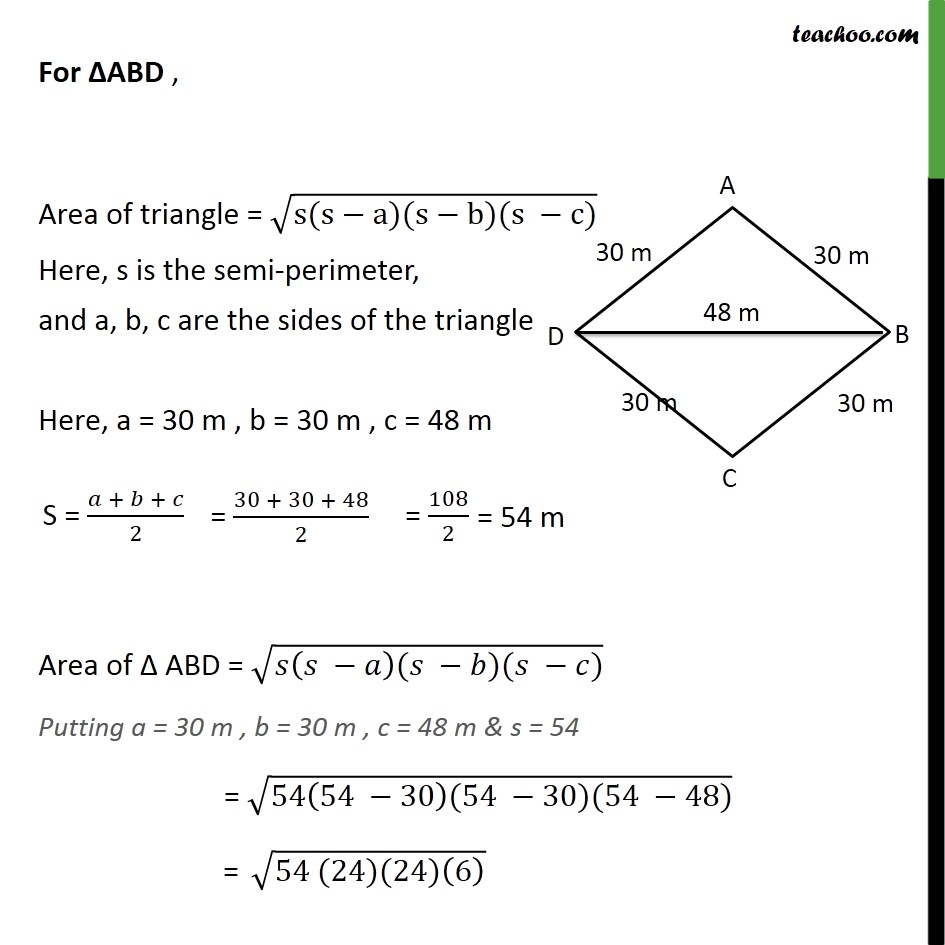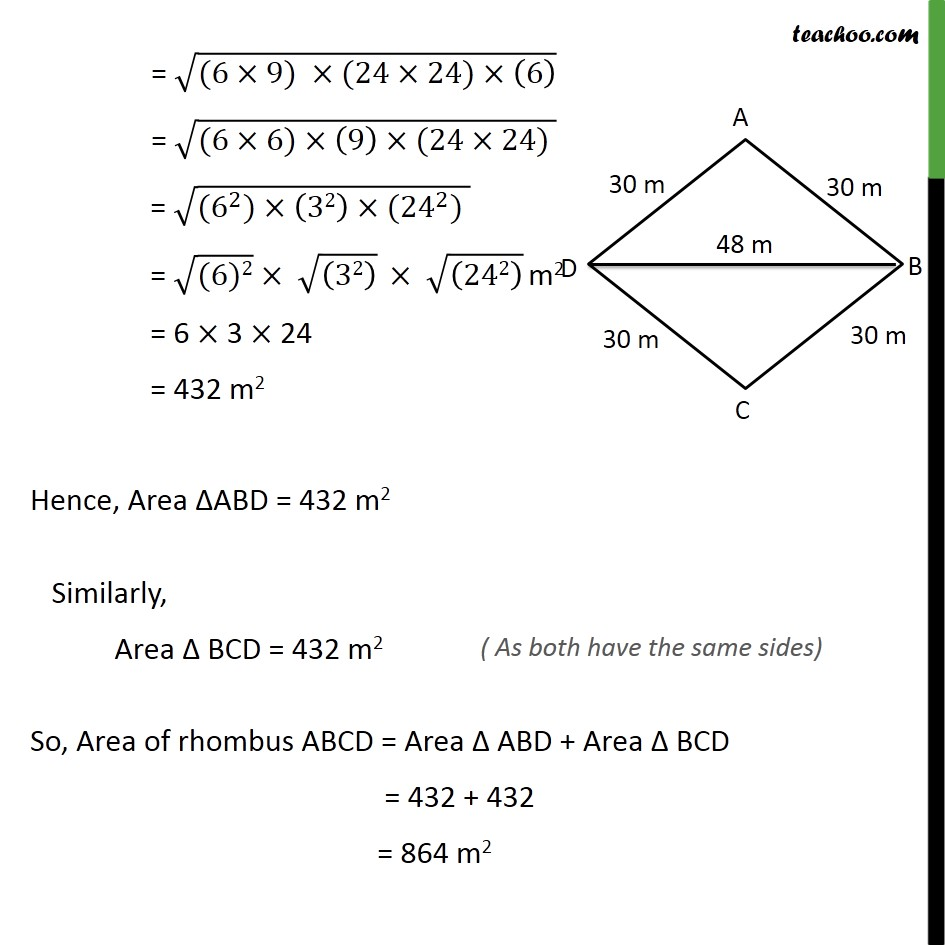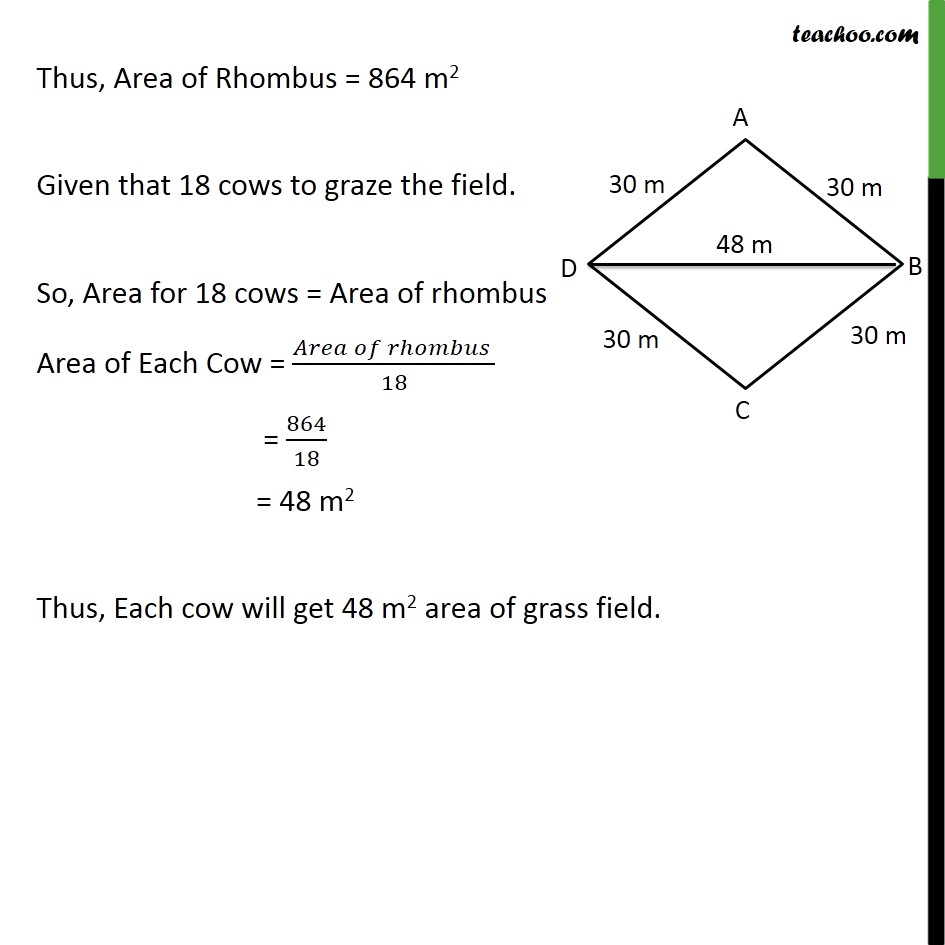1. Class 9
2. Important Questions for Exam - Class 9
3. Chapter 12 Class 9 Herons Formula

Transcript

Ex 12.2, 5 A rhombus shaped field has green grass for 18 cows to graze. If each side of the rhombus is 30 m and its longer diagonal is 48 m, how much area of grass field will each cow be getting? Let the field be ABCD It is Given that Side of rhombus = 30 m AB = BC = CD = AD = 30 m Also, Diagonal = 48 m BD = 48 m. Area of rhombus = Area ABD + Area BCD Finding area ABD For ABD , Area of triangle = (s(s a)(s b)(s c)) Here, s is the semi-perimeter, and a, b, c are the sides of the triangle Here, a = 30 m , b = 30 m , c = 48 m Area of ABD = ( ( )( )( )) Putting a = 30 m , b = 30 m , c = 48 m & s = 54 = (54(54 30)(54 30)(54 48)) = (54 (24)(24)(6) ) = ((6 9) (24 24) (6) ) = ((6 6) (9) (24 24) ) = ((6^2) (32) ( 24 ^2) ) = ((6)2) ((32) ) ((242) ) m2 = 6 3 24 = 432 m2 Hence, Area ABD = 432 m2 Similarly, Area BCD = 432 m2 So, Area of rhombus ABCD = Area ABD + Area BCD = 432 + 432 = 864 m2 Thus, Area of Rhombus = 864 m2 Given that 18 cows to graze the field. So, Area for 18 cows = Area of rhombus Area of Each Cow = ( )/18 = 864/18 = 48 m2 Thus, Each cow will get 48 m2 area of grass field.

Chapter 12 Class 9 Herons Formula

Class 9
Important Questions for Exam - Class 9

About the AuthorDavneet Singh
Davneet Singh is a graduate from Indian Institute of Technology, Kanpur. He has been teaching from the past 9 years. He provides courses for Maths and Science at Teachoo.